## 2. 6. 4 Quadrature of Discretized Functions

The field of numerical quadrature can be divided into the integration of known analytical functions, and the quadrature of element-wise given functions. Both are used in different cases. The first method is commonly used for functions, which are not integrable by analytical means. The main question is to find an accurate approximation of the integral value, usually by point-wise evaluation of the function value or the derivative. An archetypical method for such an integration is the Gauß-integration method . Such methods inherently exhibit a discretization error, which usually depends on the accuracy of the resolution of the tesselation. Finer resolutions lead to more accurate approximations of the integral.

In the second case the functions are defined explicitly, for instance as a member of the function space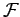. In this case all integrals can be determined explicitly. The quadrature can be carried out without a discretization error caused by the interpolation of the point-wise given function. In many cases this kind of quadrature is not used for the purpose of obtaining the integral itself but for the specification of differential equations, for instance, in finite element methods.

In this section a method to calculate the integral of a certain subdomain of the simulation domain is shown. Such an integration domain is typically tessellated into several elements, on which typically the same shape functions are defined and coefficients are stored. The function can be retrieved by inserting the coefficients into the weighting coefficients of the function.

In order to determine the integral over the integration domain, it is necessary to calculate the integral on one cell, which depends on values stored either on the respective cell itself or on subsets of the cell such as edges or vertices. The following integration is carried out for linearly bounded triangles. This method shall show the general methodology and is applicable for different shapes of elements and different shape functions, as long as the integration can be performed analytically. In other cases, methods of numerical integration have to be used for each single element. The analytical result of the integration leads to the following results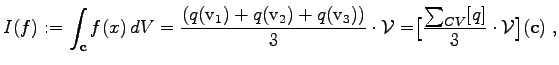(2.62)

where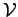denotes the volume of the cell. Combined with the formula for the value of the simplices, the formula can be generalized to simplices of arbitrary dimension.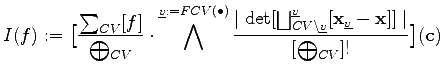(2.63)

The integration of the cell complex or a subset of the cell complex, which represents the integration domain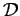can be obtained by the following formula: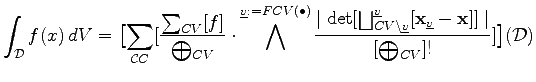(2.64)

This formula yields the integral of a piecewise-linear function, where the subdomains, on which linear functions are defined, are simplices. This holds true for arbitrary dimensions and, therefore, represents a functional formulation of a general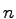-dimensional algorithm. As such, it can be directly used in a program, only syntactical changes are necessary due to the restrictions of the programming language.

Michael 2008-01-16